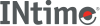﻿ frexpINtime SDK Help
frexp

Gets the mantissa and exponent of a double value.

```#include <math.h>

double frexp (double x, int *expptr);
```

#### Parameters

`x`
Value to find exponent for.
`expptr`
Pointer to stored integer exponent n.

#### Remarks

Breaks down the value `x` into a mantissa `m` and an exponent `n`, such that the absolute value of `m` is greater than or equal to 0.5 and less than 1.0, and `x` = `m` * 2`n`.

#### Return Values

• The mantissa value.
• 0 for both the mantissa and the exponent if x is 0.

#### Requirements

Versions Defined in Include Link to
INtime 3.0 intime/rt/include/math.h math.h clib.lib